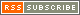# Analytical Instrument Documents## JEOL Resources

Documentation in support of your JEOL product.

## Strong interaction between light and electrons (2) "Four states of interaction between photon and spins"

A conventional ESR spectrometer uses a cavity for microwave irradiation and detection of ESR absorption. On the resonance state, it can be considered as a model that spins absorb energy of ℎ𝜈=𝑔𝜇𝐵𝐵 and then release it to the lattice system one way, where h: Planck constant, ν: frequency, g: g-value, μB: Bohr magneton, and B: magnetic flux density.
However, the interaction between photon of microwave and spins of electrons is a little more complex in fact.
Figure 1 is a modelized drawing that expresses energy flow of microwave photon and spins. The cavity resonates with angular frequency 𝜔c, relaxes with velocity of 𝜅𝑐=𝜔𝑐 𝑄𝑢 , which is inversely proportional to unloaded Q value of the cavity. On the other hand, spins do precess with an angular frequency of 𝜔𝑚𝛾𝑒 𝐵𝑚 under the static magnetic field 𝐵𝑚.
When the resonant condition of 𝜔𝑐 𝜔𝑚 is satisfied, excited electron spins that absorbed microwave energy relax with the velocity of 𝛾𝑚 (half width: half width at half maximum (HWHM)), which corresponds to spectral line width. At this time, photon and electron spins exchange energy with a coupling constant 𝑔𝑚. The coupling constant 𝑔𝑚 is expressed aswhere 𝜂𝑚𝑠𝑞𝑟𝑡 is the square root of the filling factor of the cavity, 𝛾𝑒 is gyromagnetic ratio of the electron, ℏ is reduced Planck constant (h/2π), 𝜇0 is vacuum permeability, 𝑉𝑐 is the volume of the cavity, N is number of magnetic ions, and S is spin quantum number.## Four states of interaction between photon and spins

Interaction between photon and spins can be categorized to four states according to the relation between the coupling constant (𝑔𝑚), relaxation velocity (𝜅𝑐) of photon, and relaxation velocity (𝛾𝑚) of spins.

• 『weak coupling』:
A state that corresponds to the condition of 𝑔𝑚𝜅𝑐 and 𝑔𝑚 𝛾𝑚. Normal ESR measurement is done in this state.
• 『Purcell effect』:
A state that corresponds to the condition of 𝑔𝑚 < 𝛾𝑚 and 𝑔𝑚 > 𝜅𝑐.
• 『strong coupling』:
A state that corresponds to the condition of 𝑔𝑚 > 𝛾𝑚 and 𝑔𝑚 > 𝜅𝑐. It behaves as a "quasi particle" that is unified by photon and spins.
• 『magnetically induced transparent (MIT)』:
A state that corresponds to the condition of 𝑔𝑚 < 𝜅𝑐 and 𝑔𝑚 > 𝛾𝑚.

Special care should be taken to the state of "Purcell effect" and "strong coupling", in practical ESR measurements.
Extraordinary spectral line shape, as shown in Application Note ER200006E  , might be due to the interaction between microwave photon and spins. An excess sample amount would produce an unexpected effect according to this photon- spin interaction, because equation 1 indicates that coupling constant is proportional to the square root of spin numbers. As shown in Fig. 2, resonant frequency shift of the cavity (dotted lines corresponded to AFC balance) on measuring spectrum can help to check the abnormal interaction.## Fig. 2　Frequency shift of the cavity on measuring ESR spectra (conf. Application Note ER200006E ).

(a) Normal (Set_B). AFC balance does not almost move. (b) Excess sample (Set_A). AFC balance moves hard.

Investigating the frequency shift of the cavity around the resonant region, we can estimate what kind of interaction the system can be categorized to. Figure 3 is a mapping graph that the frequency spectra of the cavity ("Set_A" configuration as shown in Application Note ER200006E) is arrayed on the respective magnetic fields. Based on the simulation using the equation of Q-dip (S11 parameter) shown in references, coupling constant was estimated to ca 1.2 MHz. This situation is inferred to the state of "Purcell effect", because it is under the condition of 𝑔𝑚 / 2𝜋 < 𝛾𝑚 / 2𝜋 and 𝑔𝑚 / 2𝜋 > 𝜅𝑐 / 2𝜋 , where spectral half width (HWHM（half width at half maximum) is ca 121 μT (𝛾𝑚 / 2𝜋=3.39 MHz), Qu value of the cavity is ca 18,000 (𝜅𝑐 / 2𝜋=0.52 MHz). Such a situation, normal ESR spectra can not be obtained. Especially, using high Q cavity that includes an excess sample might induce "Purcell effect", and it should be taken care of in the interaction. Furthermore, when the sample is not a paramagnet, but a ferromagnet, and its line width is narrow, we might encounter the state of "strong coupling" (continued to Application Note ER200008E ).## Fig.3　Field dependence of the frequency spectra (Q-dips).

(a) Observed. (b) Simulation results based on the equation in the literature.

Reference:  X. Zhang, C-L. Zou, L. Jiang, and H. X. Tang, Phys. Rev. Lett. 113, 156401 (2014).
 E. Abe, H. Wu, A. Ardavan, and J. J. L. Morton,　Appl. Phys. Lett. 98, 251108 (2011).
 Patent, US10288707B2 "Relaxation time measuring method and magnetic resonance measuring apparatus".